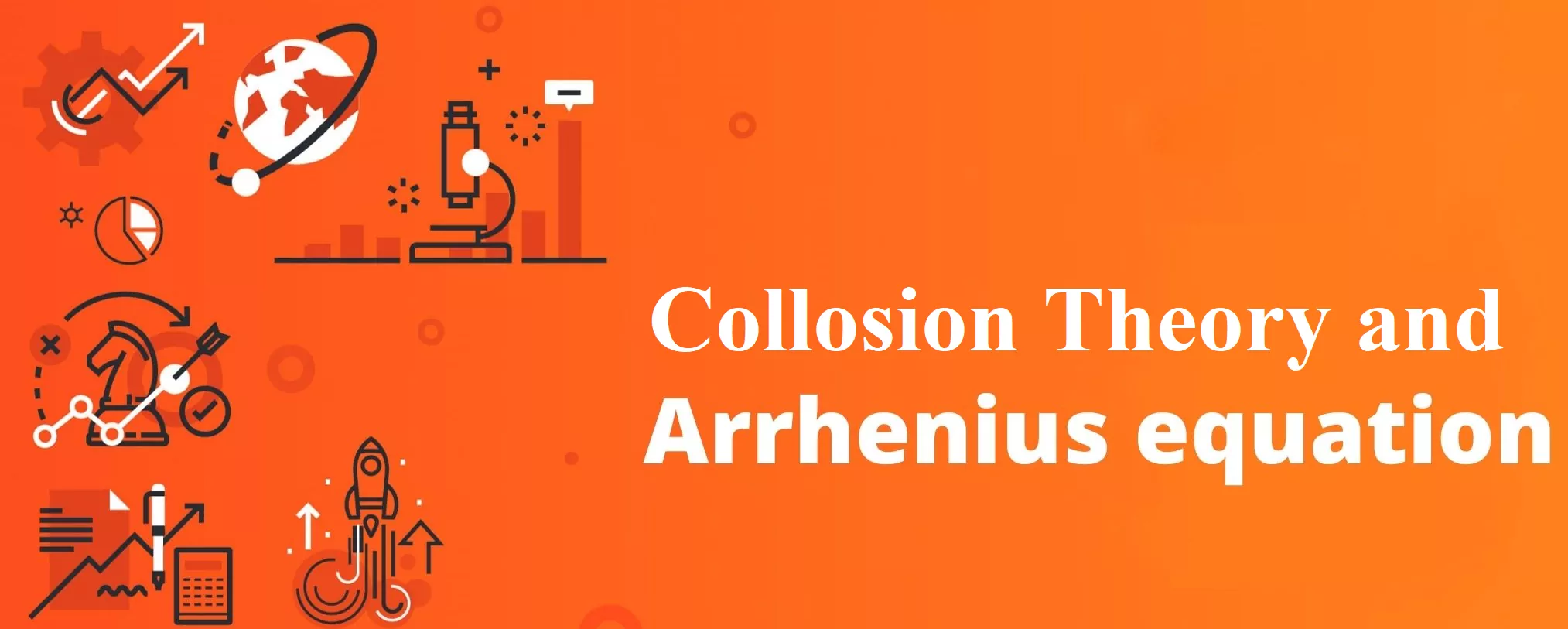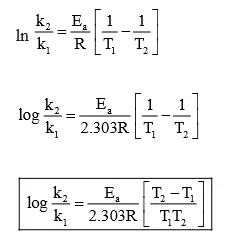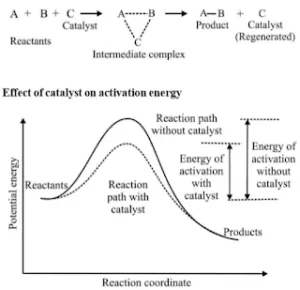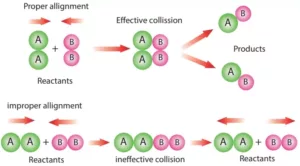# Collision Theory and Arrhenius EquationCollision Theory and Arrhenius Equation

Arrhenius equation:
Quantitatively, the temperature dependence of the rate of a chemical reaction can be explained by Arrhenius equation
k = A e – Ea /RT
where A is the Arrhenius factor or the frequency factor or the pre-exponential factor. R is gas constant and E a is activation energy measured in joules/mole.
The factor e – Ea /RT corresponds to the fraction of molecules that have kinetic energy greater than Ea.
Thus, it has been found from the Arrhenius equation that increasing the temperature or decreasing the activation energy will result in an increase in the rate of the reaction and an exponential increase in the rate constant.
Taking the natural logarithm of both sides of the equation
ln K = – E + ln A
RT
At temperature T1, equation
ln K1 =  – E + ln A
RT1
At temperature T2, equation
ln K2 = –  E + ln A
RT2
(since A is constant for a given reaction)
K1 and K2  are the values of rate constants at temperatures T1 and T2 respectively.
Subtracting equation form, we obtain
ln K2 – ln K1 =    E  –   E
RT1     RT2Collision Theory and Arrhenius Equation

Effect of Catalyst: A catalyst is a substance that alters the rate of a reaction without itself undergoing any permanent chemical change. The action of the catalyst can be explained by intermediate complex theory. According to this theory, a catalyst participates in a chemical reaction by forming an intermediate complex. This is unstable and decomposes to yield products and the catalyst.Collision Theory and Arrhenius Equation

A catalyst increases the rate of a chemical reaction by providing an alternate pathway or reaction mechanism by reducing the activation energy between reactants and products. The important characteristics of a catalyst are:

1. A small amount of the catalyst can catalyze a large number of reactants.
2. A catalyst does not alter Gibb’s energy, ΔG of a reaction. It catalyzes spontaneous reactions but does not catalyze non-spontaneous reactions.
3. A catalyst does not change the equilibrium constant of a reaction, but it helps to attain the equilibrium faster by increasing the rate of both forward as well as backward reactions.

Collision Theory and Arrhenius Equation

Collision Theory: This theory was developed by Max Trautz and William Lewis. It is based on the kinetic theory of gases. According to this theory, the reactant molecules are assumed to be hard spheres, and reaction has occurred when molecules collide with each other. The number of collisions per second per unit volume of the reaction mixture is known as collision frequency (Z).Another factor that affects the rate of chemical reactions is the activation energy.
For a bimolecular elementary reaction
A + B → Products
The rate of reaction can be expressed as
Rate (r)= ZAB e – Ea /RT
Where ZAB represents the collision frequency of reactants, A and B, and e– Ea/RT represents the fraction of molecules with energies equal to or greater than EaComparing with the Arrhenius equation, we can see that A is related to collision frequency.
A third factor that affects the rate of a chemical reaction is the proper orientation. To account for this, a factor P called the Probability or Steric factor is introduced. So the above equation becomes:

Rate (r)= PZAB e – Ea /RT

Collision Theory and Arrhenius Equation